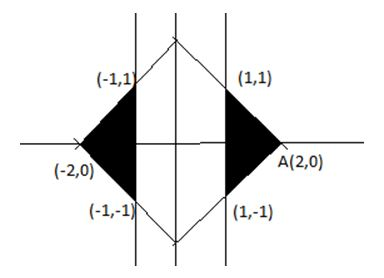# Let S be the set of all points (x, y) - Quantitative Aptitude – Modern Maths | Coordinate Geometry |

## CAT 2019 – Slot 1 - Coordinate Geometry - Question 1 - Let S be the set of all points (x, y)

Q. 1: Let S be the set of all points (x, y) in the x-y plane such that | x | + | y | ≤ 2 and | x | ≥ 1. Then, the area, in square units, of the region represented by S equals

The graph of the two functions can be drawn as below:Area of the shaded region = 2*(area of one shaded region) = 2*1/2 *1*2 = 2 unit

### Past Year Question Paper & SolutionsCounselling Session
By IIM Mentor
##### Checkout Other Questions of CAT 2019 Slot 1 Paper:

Verbal Ability :              |   Q.01- Q.05  |  Q.06- Q.10  |  Q.11- Q.15  |  Q.16- Q.20  |  Q.21- Q.24  |  Q.25- Q.29  |  Q.30 – Q.34  |

Logical Reasoning :    |   Q.29 – Q.32  |

Quantitative Aptitude: |

### Free Material Area